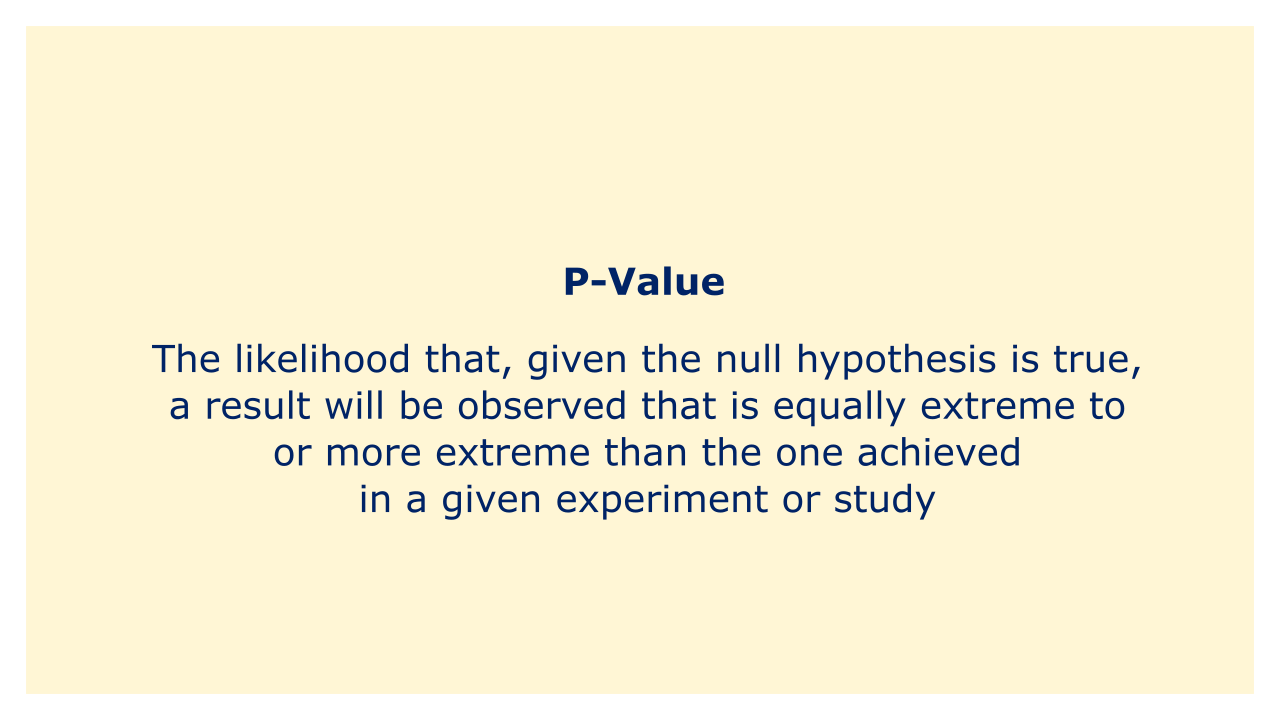# P-ValueImage: Moneybestpal.com

### A statistical measure known as a p-value is frequently employed in hypothesis testing to assess the likelihood that a specific outcome was the result of pure chance. In further detail, it is the likelihood that, given the null hypothesis is true, a result will be observed that is equally extreme to or more extreme than the one achieved in a given experiment or study.

The null hypothesis states that there is no association between two variables or that there is no significant difference between two groups. Researchers build up a null hypothesis and an alternative hypothesis, which is the antithesis of the null hypothesis, while conducting a hypothesis test. The null hypothesis is subsequently rejected in favor of the alternative hypothesis if the estimated p-value is less than a preset threshold (often 0.05) based on the observed data.

Consider a scenario where a researcher wishes to determine whether a novel medication may lower blood pressure better than a placebo. They established the alternative hypothesis, which is that the medication is successful, and the null hypothesis, according to which there is no difference in blood pressure between the two groups. They do research and come up with a p-value of 0.02, which is below the 0.05 cutoff. This shows that there is sufficient data to reject the null hypothesis and draw the conclusion that the medication lowers blood pressure effectively.

It is important to remember that the p-value does not reflect the magnitude of the effect or the clinical importance of the finding. If the null hypothesis is correct, it just informs us of the likelihood of getting the observed result. In order to completely comprehend the findings of a study, it should be used in conjunction with other metrics like effect size and confidence intervals. Also, because sample size, study design, and other variables might alter the p-value, it should not be the only criterion used to assess the significance of a result.
Tags